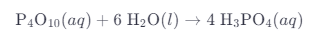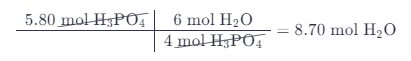# Tetraphosphorus Decoxide Reacts With Water To Produce Phosphoric Acid

We thoroughly check each answer to a question to provide you with the most correct answers. Found a mistake? Let us know about it through the REPORT button at the bottom of the page.

Tetraphosphorus decoxide (P4O10) reacts with water to produce phosphoric acid. Write and balance the equation for this reaction, and determine the number of moles of each reactant required to produce 5.80 moles of phosphoric acid.

Write a balanced equation for this reaction:1. Begin with the number of moles of H3PO4.
2. Use a mole ratio to convert moles of H3PO4 into moles of P4H10.1. Begin with the number of moles of H3PO4.
2. Use a mole ratio to convert moles of H3PO4 into moles of H20.1.45 mol P4O10 and 8.70 mol H20.